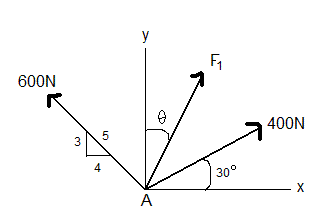# Finding the Magnitude and Direction of Force one with the Resultant Given

## Homework Statement

Determine the magnitude and direction theata of F1 so that the resultant force is directed vertically upward and has a magnitude of 800N.

## Homework Equations## The Attempt at a Solution

I have difficulty of where to start the problem? do i sum up the forces of the x axis with unknowns in it and forces of the y axis with the unknown forces...where should i start....and how do i start solving the problem????

Please someone help me with this....

Thanks,

Nacha

tiny-tim
Homework Helper
Welcome to PF!

I have difficulty of where to start the problem? do i sum up the forces of the x axis with unknowns in it and forces of the y axis with the unknown forces...where should i start....and how do i start solving the problem????

Hi Nacha ! Welcome to PF!Yes … split the force F1 into Fx and Fy

then do x and y separately …

finally combine Fx and Fy to give you F1 and theta.Hi,

Thanks for helping me, but one more question, my Fx and Fy for F1 is unkown as shown in the diagram that i have posted earlier, so how do i go about solving for the magnitude of F1 and the direction of F1. Sorry for the trouble....

thanks
Nacha

tiny-tim
Homework Helper
Hi,

Thanks for helping me, but one more question, my Fx and Fy for F1 is unkown as shown in the diagram that i have posted earlier, so how do i go about solving for the magnitude of F1 and the direction of F1. Sorry for the trouble....

thanks
Nacha

Hi,

Have you got Fx and Fy yet?

You can get them from the diagram.

Then use Pythagoras, and tangents.can anyone explain how to solve this problem in complete details? i don't understand the explanations. i have the same problem too.tiny-tim
Homework Helper
welcome to pf!

hi sandra! welcome to pf!you need to get all the y-components adding to 800, and all the x-components adding to zero

that will give you two equations involving two unknowns (F1 and θ), which should enable you to find the unknowns

what do you get?owhh.. how about to solve this question:

determine the magnitude and direction of P so that the resultant of P and the 900N force is a vertical force of 2700N directed downward.

is it using the same method also? it's almost the same right?

tiny-tim
Homework Helper
right!thanks so much tiny-tim! i'm doing my engineering mechanics tutorial problems right now.. if i have any problem again, i'll ask your help later.. ;)

err... do you online often? then, i'll know when can i ask for your help..

tiny-tim
Homework Helper
sandra, just post a new thread any timesomewhere in the world, there'll be someone awake and logged in ready to help, whatever time it istiny-tim

okie! will do..i got a similar problem but i dont have a resultant force :\ not sure on how to solve ive found theata using a parallelogram
theata is the one between the X axis and F1

#### Attachments

• untitled.bmp
115.6 KB · Views: 842
tiny-tim
Homework Helper
Welcome to PF!

Hi Zlash! Welcome to PF!(have a theta: θ)

Sorry, I dont understand the question …

you have three forces (100N 350N and R), and also a line called X at 30º …

if X is equivalent to F1 in Nacha's question, then changing the magnitude of X will give different directions for the resultant R (but a unique magnitude for each direction) …

if neither the magnitude nor the direction of R is given, I don't see how you can answer the questionHi Zlash! Welcome to PF!(have a theta: θ)

Sorry, I dont understand the question …

you have three forces (100N 350N and R), and also a line called X at 30º …

if X is equivalent to F1 in Nacha's question, then changing the magnitude of X will give different directions for the resultant R (but a unique magnitude for each direction) …

if neither the magnitude nor the direction of R is given, I don't see how you can answer the questionnah X' is a vector that cuts through.
in the quiz im doing it doesnt say anything about a resultant but im assuming we have to use the one given in the text book. so the resultant measured from the positive X' -the line- is 600N
i found θ as 60. θ being between F1 and the X axis( not x' )
im not sure how i can work through forces based on different axis kinda messes me up =/
im trying it now

Last edited:
hi all
∑F x= 400cos30 – 600 cos36.87 + F1 sinØ =0
∑F y= 400sin30 – 600sin36.87 + F1 cosØ =0
R=√(〖fx〗^2+〖fy〗^2 )
800=√((〖298.35+f1sinØ)〗^2+〖(560+f1 cosØ)〗^2 )

As we see ; we have two equation and two unknown so we can get the magnitude and direction of f1